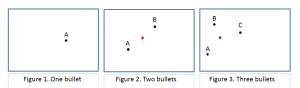30
Aug 16

## The pearls of AP Statistics 24

Unbiasedness: the stumbling block of a Statistics course

### God is in the detail

They say: A good estimator has a sampling distribution that is centered at the parameter. We define center in this case as the mean of that sampling distribution. An estimator with this property is said to be unbiased. From Section 7.2, we know that for random sampling the mean of the sampling distribution of the sample mean x equals the population mean μ. So, the sample mean x is an unbiased estimator of μ. (Agresti and Franklin, p.351).

I say: This is a classic case of turning everything upside down, and this happens when the logical chain is broken. Unbiasedness is one of the pillars of Statistics. It can and should be given right after the notion of population mean is introduced. The authors make the definition dependent on random sampling, sampling distribution and a whole Section 7.2. Therefore I highly doubt that any student can grasp the above definition. My explanation below may not be the best; I just want to prompt the reader to think about alternatives to the above "definition".

### Population mean versus sample mean

By definition, in the discrete case, a random variable is a table values+probabilities:

 Values Probabilities$X_1$$p_1$ ... ...$X_n$$p_n$

If we know this table, we can define the population mean$\mu=EX=p_1X_1+...+p_nX_n$. This is a weighted average of the variable values because the probabilities are percentages:$0 for all$i$ and$p_1+...+p_n=1$. The expectation operator$E$ is the device used by Mother Nature to measure the average, and most of the time she keeps hidden from us both the probabilities and the average$EX.$

Now suppose that$X_1,...,X_n$ represent a sample from the given population (and not the values in the above table). We can define the sample mean$\bar{X}=\frac{X_1+...+X_n}{n}$. Being a little smarter than monkeys, we instead of unknown probabilities use the uniform distribution$p_i=1/n$. Unlike the population average, the sample average is always possible to calculate, as long as the sample is available.

Consider a good shooter shooting at three targets using a good rifle.The black dots represent points hit by bullets on three targets. In Figure 1, there was only one shot. What is your best guess about where the bull's eye is? Regarding Figure 2, everybody says that probably the bull's eye is midway (red point) between points A and B. In Figure 3, the sample mean is represented by the red point. Going back to unbiasedness: 1) the bull's eye is the unknown population parameter that needs to be estimated, 2) points hit by bullets are sample observations, 3) their sample mean is represented by red points, 4) the red points estimate the location of the bull's eye. The sample mean is said to be an unbiased estimator of population mean because

(1)$E\bar{X}=\mu$.

In words, Mother Nature says that, in her opinion, on average our bullets hit the bull's eye.

This explanation is an alternative to the one you can see in many books: in the long run, the sample mean correctly estimates the population mean. That explanation in fact replaces equation (1) by the corresponding law of large numbers. My explanation just underlines the fact that there is an abstract average, that we cannot use, and the sample average, that we invent to circumvent that problem.

See related theoretical facts here.